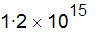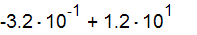﻿ Scientific Notation

# Scientific Notation

Top  Previous  Next
 The equation tool can automatically format scientific notation.  The capital E followed by a number will be transformed into ×10 to the power of the number.   The equation tool will correctly format numbers with negative exponents.   The equation tool will use the currently selected multiplication symbol (× or ⋅).   Examples:is entered as 1.2E15is entered as -3.2E-1+1.2E1